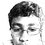# An Interesting Conclusion

It is a known fact that powers of $2$ add upto any natural sum less than the next power of $2$. This has been the basis for many practical problems with measurements, and allows us to count binary. The notation of this property is:

$(\displaystyle\sum_{i=0}^{n} 2^{i}) + 1 = 2^{n+1}$

Then I realised, this could be extended to powers of $3$, after the sum is multiplied by $2$ i.e.

$2(\displaystyle\sum_{i=0}^{n} 3^{i}) + 1 = 3^{n+1}$

This worked even for $4$, when the sum was multiplied by $3$

$3(\displaystyle\sum_{i=0}^{n} 4^{i}) + 1 = 4^{n+1}$

Thus, I thought I may generalize it:

$(x-1)(\displaystyle\sum_{i=0}^{n} x^{i}) + 1 = x^{n+1}$, for all $x\in\mathbb{N}$

Is this generalization correct, or is there a limit to $x$?

If there is a proof for this statement, can someone post it below?Note by Nanayaranaraknas Vahdam
7 years, 2 months ago

This discussion board is a place to discuss our Daily Challenges and the math and science related to those challenges. Explanations are more than just a solution — they should explain the steps and thinking strategies that you used to obtain the solution. Comments should further the discussion of math and science.

When posting on Brilliant:

• Use the emojis to react to an explanation, whether you're congratulating a job well done , or just really confused .
• Ask specific questions about the challenge or the steps in somebody's explanation. Well-posed questions can add a lot to the discussion, but posting "I don't understand!" doesn't help anyone.
• Try to contribute something new to the discussion, whether it is an extension, generalization or other idea related to the challenge.

MarkdownAppears as
*italics* or _italics_ italics
**bold** or __bold__ bold
- bulleted- list
• bulleted
• list
1. numbered2. list
1. numbered
2. list
Note: you must add a full line of space before and after lists for them to show up correctly
paragraph 1paragraph 2

paragraph 1

paragraph 2

[example link](https://brilliant.org)example link
> This is a quote
This is a quote
    # I indented these lines
# 4 spaces, and now they show
# up as a code block.

print "hello world"
# I indented these lines
# 4 spaces, and now they show
# up as a code block.

print "hello world"
MathAppears as
Remember to wrap math in $$ ... $$ or $ ... $ to ensure proper formatting.
2 \times 3 $2 \times 3$
2^{34} $2^{34}$
a_{i-1} $a_{i-1}$
\frac{2}{3} $\frac{2}{3}$
\sqrt{2} $\sqrt{2}$
\sum_{i=1}^3 $\sum_{i=1}^3$
\sin \theta $\sin \theta$
\boxed{123} $\boxed{123}$

Sort by:

I actually registered this about a year ago but couldn't find a proof. Tag me if you can.

- 7 years, 2 months ago

Tag? How?

- 7 years, 2 months ago

Like this: @Sharky Kesa

- 7 years, 2 months ago

Easy proof.

Let S = sum_i=1^n (x^i). Take -1 from both sides. After multiplying x-1 into the LHS sum and a bit of manipulation, it is easy to see LHS = (x^n+1) + S - S - 1 = RHS.

Thus, we can also conclude that x can be any complex number.

- 7 years, 2 months ago

Thank you for the proofs! Now that this has been proven, can it be altered to give the value of any number $x^{y}$

- 7 years, 2 months ago

It's pretty easy.Think of it as a polynomial

$x^{n-1}+x^{n-2}+...+1$

Then multiply by $x-1$ and you will simply get (as things cancel)

$x^n-1$

- 7 years, 2 months ago

I just realised that. Me and my slow head.

- 7 years, 2 months ago